$\newcommand{\R}{\mathbb{R}}$ $\newcommand{\E}{\mathbb{E}}$ $\newcommand{\Var}{\mathrm{Var}}$ $\newcommand{\ve}{\varepsilon}$ $\newcommand{\bone}{ \boldsymbol{1} }$ $\newcommand{\bzero}{ \boldsymbol{0} }$ $\newcommand{\bmu}{ \boldsymbol{\mu} }$ $\newcommand{\bu}{ \boldsymbol{u} }$ $\newcommand{\bv}{ \boldsymbol{v} }$ $\newcommand{\bx}{ \boldsymbol{x} }$ $\newcommand{\by}{ \boldsymbol{y} }$ $\newcommand{\bw}{ \boldsymbol{w} }$ $\newcommand{\bwhat}{\widehat{\bw}}$ $\DeclareMathOperator*{\argmin}{argmin}$ $\newcommand{\norm}{ \left\|{#1}\right\| }$

# Linear Regression¶

In linear regression our predictors are linear functions $f : \R^d\to\R$ each parameterized by a vector $\bw\in\R^d$ real coefficients. That is, $f(\bx) = \bw^{\top}\bx$.

Given a training set $(\bx_1,y_1),\ldots,(\bx_m,y_m) \in \R^d\times\R$, the linear regression predictor is the Empirical Risk Minimizer with respect to the square loss, $$\bwhat = \argmin_{\bw\in\R^d} \sum_{t=1}^m \big(\bw^{\top}\bx_t - y_t\big)^2$$ Now let $\bv = \big(\bw^{\top}\bx_1,\ldots,\bw^{\top}\bx_m\big)$ and $\by = (y_1,\ldots,y_m)$. Then $$\sum_{t=1}^m \big(\bw^{\top}\bx_t - y_t\big)^2 = \norm{\bv-\by}^2.$$ Since $\bv = S\bw$, where $S$ is a $m \times d$ matrix such that $S^{\top} = [\bx_1,\ldots,\bx_m]$, we may also write $$\bwhat = \argmin_{\bw\in\R^d} \norm{S\bw-\by}^2$$ Since $F(\bw) = \norm{S\bw-\by}^2$ is a convex function, the minimizer satisfies the condition $\nabla F(\bw) = \bzero$.

Using matrix calculus, we have that $\nabla\norm{S\bw-\by}^2 = 2S^{\top}(S\bw-\by)$. Hence, $\nabla\norm{S\bw-\by}^2 = \bzero$ for $\bw = \big(S^{\top}S\big)^{-1}S^{\top}\by$ provided $S^{\top}S$ is invertible.

Therefore, whenever $S^{\top}S$ is invertible we have that $\bwhat = \big(S^{\top}S\big)^{-1}S^{\top}\by$.

Since the Bayes optimal predictor for square loss is $f(\bx) = \E[Y \mid \bx]$, the Bayes optimal predictor is a linear function when $y = \bu^{\top}\bx + \ve_{\bx}$, where $\ve_{\bx}$ is an independent random variable such that $\E[\ve_{\bx} \mid \bx] = 0$.

In :
import pandas as pd
import numpy as np
import matplotlib
import matplotlib.pyplot as plt

import seaborn as sns

from sklearn import decomposition
from sklearn.linear_model import LinearRegression, Ridge
from sklearn.model_selection import train_test_split, learning_curve, cross_val_score, validation_curve, GridSearchCV
from sklearn.metrics import mean_squared_error
from sklearn.datasets import make_regression
from sklearn.preprocessing import PolynomialFeatures
from sklearn.pipeline import make_pipeline

#from auxCode import *
%matplotlib inline


We start by using the function make_regression() to generate a random dataset on which the Bayes optimal predictor is a linear function. We set $d=1$, $m=1000$, and $\sqrt{\Var[\ve_{\bx} \mid \bx]} = 15$.

In :
X, y, w = make_regression(n_samples=1000, n_features=1, n_informative=1, n_targets=1,
noise=15.0, shuffle=True, coef=True, random_state=42)


We can inspect the coefficient of the Bayes optimal predictor.

In :
np.round(w.item(), decimals=2)

Out:
16.75

The Bayes risk is the squared conditional variance, averaged over data points. In our case, Bayes risk $(15)^2 = 225$.

If we compute it on our dataset we get $220.6$, which is reasonable considering we have sampled just $1000$ data points.

In :
y_opt = w.item()*X
np.round(mean_squared_error(y, y_opt), decimals=2)

Out:
220.57

Next, we split the dataset in train and test, with proportions $60\%$ and $40\%$.

In :
X_train, X_test, y_train, y_test = train_test_split(X, y, test_size=0.4, random_state=42)


We run a linear regression learner on the training data and print the learned coefficient.

In :
learner = LinearRegression()
learner.fit(X_train, y_train)
np.round(learner.coef_, decimals=2)

Out:
17.02

Note that the learned linear regressor is pretty close to the coefficient $16.75$ of the Bayes optimal predictor.

We then compute test predictions and measure test error with respect to the square loss.

In :
y_pred = learner.predict(X_test)
np.round(mean_squared_error(y_test, y_pred), decimals=2)

Out:
230.33

If we plot the test points and the two linear regressors we can get an idea of how good is linear regression when the Bayes optimal predictor is also a linear function.

In :
x_min = np.min(X)
x_max = np.max(X)
v = np.arange(x_min, x_max, 0.2)

plt.scatter(X_test, y_test,  color='black')
plt.plot(X_test, y_pred, color='blue', linewidth=2)
plt.plot(v, v*w.item(), color='red', linewidth=2)
plt.show()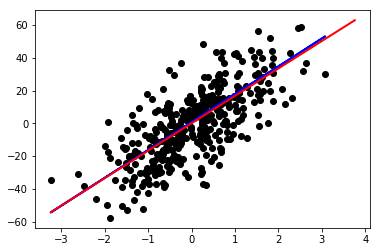The test error of the Bayes optimal predictor is only slightly lower than that of the linear regressor learned on the training set.

In :
y_opt = w.item()*X_test
np.round(mean_squared_error(y_test, y_opt), decimals=2)

Out:
227.94

Now we keep the same training set and change the labels to make the Bayes optimal predictor a nonlinear function of $x$, $y = \sin(x) + 0.3\ve_x$, where $\ve_x$ is an independent Gaussian random variable with mean zero and unit variance.

In :
y = X * np.sin(X) + 0.3*np.random.randn(1000,1)

In :
plt.scatter(X, y,  color='black')
plt.show()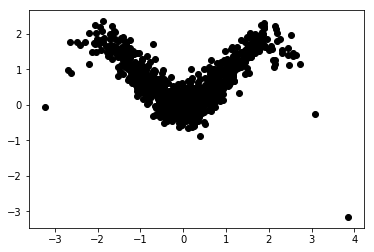If we train on the entire dataset, linear regression achieves a training error of $0.45$.

In :
learner.fit(X, y)
y_pred = learner.predict(X)
np.round(mean_squared_error(y, y_pred), decimals=2)

Out:
0.43

We now use a trick to make linear regression more powerful at the expense of increasing the number of features.

The function PolynomialFeatures() replaces the original features $\bx = (x_1,\ldots,x_d)$ with all polynomial combinations of features with degree less than or equal to a specified degree $n$. For instance, if $d=2$ and $n=2$, the original features $(x_1,x_2)$ are replaced by $(1,x_1,x_2,x_1x_2,x_1^2,x_2^2)$. Note that the number of new features is of order $d^n$.

With $n=5$ the training error of linear regression goes down to $0.10$.

In :
poly = PolynomialFeatures(degree=5, interaction_only=False, include_bias=True)
X_poly = poly.fit_transform(X)
learner.fit(X_poly, y)
y_pred = learner.predict(X_poly)

In :
np.round(mean_squared_error(y, y_pred), decimals=2)

Out:
0.09
In :
plt.scatter(X, y,  color='black')
plt.scatter(X, y_pred,  color='red')
plt.show()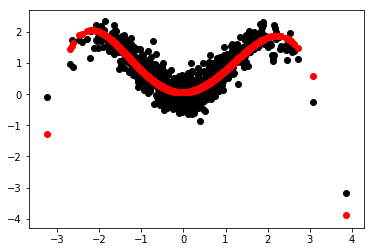We are now ready to try out linear regression on a real-world dataset.

The Boston Housing Dataset originates from the UCI Machine Learning Repository. The data was collected in $1978$ and each of the $506$ entries represent aggregated data about $14$ features for homes from various suburbs in Boston, Massachusetts. The label is the column MEDV.

We load the dataset and add column names. Note the the field separator in the file is whitespace instead of comma.

In :
names = ['CRIM', 'ZN', 'INDUS', 'CHAS', 'NOX', 'RM', 'AGE', 'DIS', 'RAD', 'TAX', 'PTRATIO', 'B', 'LSTAT', 'MEDV']
housing = pd.read_csv("Datasets/housing.csv", delim_whitespace=True, names=names)
housing.info()

<class 'pandas.core.frame.DataFrame'>
RangeIndex: 506 entries, 0 to 505
Data columns (total 14 columns):
CRIM       506 non-null float64
ZN         506 non-null float64
INDUS      506 non-null float64
CHAS       506 non-null int64
NOX        506 non-null float64
RM         506 non-null float64
AGE        506 non-null float64
DIS        506 non-null float64
RAD        506 non-null int64
TAX        506 non-null float64
PTRATIO    506 non-null float64
B          506 non-null float64
LSTAT      506 non-null float64
MEDV       506 non-null float64
dtypes: float64(12), int64(2)
memory usage: 55.4 KB

In :
housing.describe()

Out:
CRIM ZN INDUS CHAS NOX RM AGE DIS RAD TAX PTRATIO B LSTAT MEDV
count 506.000000 506.000000 506.000000 506.000000 506.000000 506.000000 506.000000 506.000000 506.000000 506.000000 506.000000 506.000000 506.000000 506.000000
mean 3.613524 11.363636 11.136779 0.069170 0.554695 6.284634 68.574901 3.795043 9.549407 408.237154 18.455534 356.674032 12.653063 22.532806
std 8.601545 23.322453 6.860353 0.253994 0.115878 0.702617 28.148861 2.105710 8.707259 168.537116 2.164946 91.294864 7.141062 9.197104
min 0.006320 0.000000 0.460000 0.000000 0.385000 3.561000 2.900000 1.129600 1.000000 187.000000 12.600000 0.320000 1.730000 5.000000
25% 0.082045 0.000000 5.190000 0.000000 0.449000 5.885500 45.025000 2.100175 4.000000 279.000000 17.400000 375.377500 6.950000 17.025000
50% 0.256510 0.000000 9.690000 0.000000 0.538000 6.208500 77.500000 3.207450 5.000000 330.000000 19.050000 391.440000 11.360000 21.200000
75% 3.677082 12.500000 18.100000 0.000000 0.624000 6.623500 94.075000 5.188425 24.000000 666.000000 20.200000 396.225000 16.955000 25.000000
max 88.976200 100.000000 27.740000 1.000000 0.871000 8.780000 100.000000 12.126500 24.000000 711.000000 22.000000 396.900000 37.970000 50.000000
In :
housing.head()

Out:
CRIM ZN INDUS CHAS NOX RM AGE DIS RAD TAX PTRATIO B LSTAT MEDV
0 0.00632 18.0 2.31 0 0.538 6.575 65.2 4.0900 1 296.0 15.3 396.90 4.98 24.0
1 0.02731 0.0 7.07 0 0.469 6.421 78.9 4.9671 2 242.0 17.8 396.90 9.14 21.6
2 0.02729 0.0 7.07 0 0.469 7.185 61.1 4.9671 2 242.0 17.8 392.83 4.03 34.7
3 0.03237 0.0 2.18 0 0.458 6.998 45.8 6.0622 3 222.0 18.7 394.63 2.94 33.4
4 0.06905 0.0 2.18 0 0.458 7.147 54.2 6.0622 3 222.0 18.7 396.90 5.33 36.2

We take a look at the distribution of the labels using the distplot() function of Seaborn.

In :
sns.distplot(housing['MEDV']);
fig = plt.figure()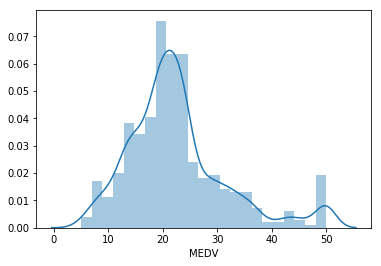<Figure size 432x288 with 0 Axes>

We also look at the correlation matrix between features. The DataFrame method corr() of Pandas computes the Pearson correlation coefficient of the sample, defined for each pair $i,j$ of features by $$r_{i,j} = \frac{\sum_{t=1}^m (x_{i,t}-\mu_i)(x_{j,t}-\mu_j)}{\sqrt{\sum_{t=1}^m (x_{i,t}-\mu_i)^2}\sqrt{\sum_{t=1}^m (x_{j,t}-\mu_j)^2}}$$ where $\mu_i = (x_{i,1}+\cdots+x_{i,m})/m$.

Recall that the Pearson correlation coefficient $r_{i,j}$ is always in the $[-1,+1]$ interval. Values of $r_{i,j}$ closer to the extremes indicate that $i$ and $j$ tend to be linearly correlated, whereas values of $r_{i,j}$ indicate that $i$ and $j$ are nearly independent.

In :
corr=housing.corr()
plt.figure(figsize=(20, 20))
sns.heatmap(corr, vmax=.8, linewidths=0.01,
square=True,annot=True,cmap='YlGnBu',linecolor="white")
plt.title('Correlation between features');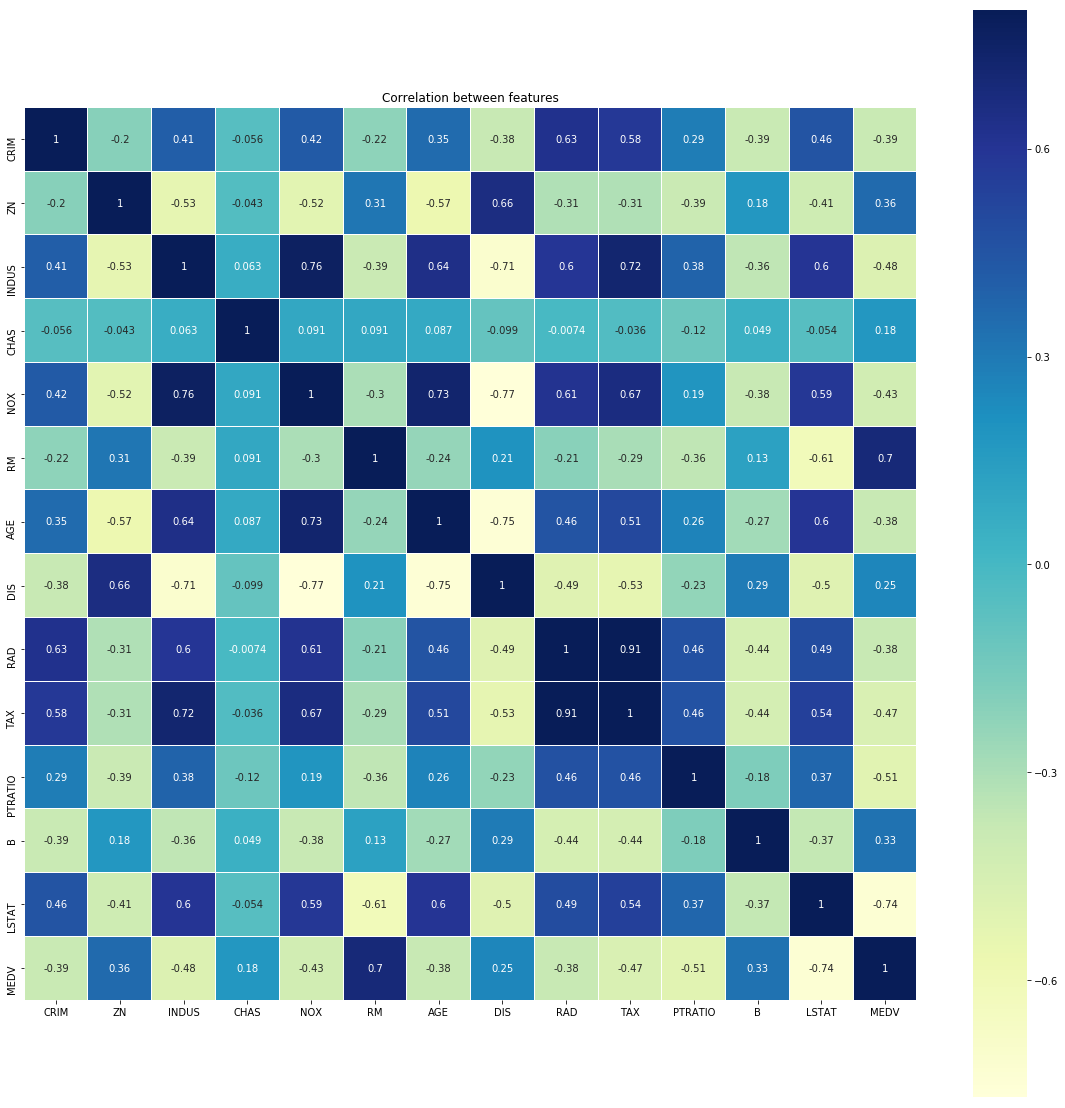Pairs of features with correlation coefficient close to $-1$ or $+1$ are redundant (for example, TAX and RAD in this dataset), and we could only keep one for each pair.

We check which feature has a correlation larger than $0.75$ in absolute value which at least some other feature.

In :
corr[((corr > 0.75) | (corr < -0.75)) & (corr != 1.0)].dropna(axis='index', how='all')

Out:
CRIM ZN INDUS CHAS NOX RM AGE DIS RAD TAX PTRATIO B LSTAT MEDV
INDUS NaN NaN NaN NaN 0.763651 NaN NaN NaN NaN NaN NaN NaN NaN NaN
NOX NaN NaN 0.763651 NaN NaN NaN NaN -0.76923 NaN NaN NaN NaN NaN NaN
DIS NaN NaN NaN NaN -0.769230 NaN NaN NaN NaN NaN NaN NaN NaN NaN
RAD NaN NaN NaN NaN NaN NaN NaN NaN NaN 0.910228 NaN NaN NaN NaN
TAX NaN NaN NaN NaN NaN NaN NaN NaN 0.910228 NaN NaN NaN NaN NaN

It turns out that we can safely drop NOX and RAD.

In :
X = housing.drop(columns=['NOX', 'RAD', 'MEDV']).values
y = housing['MEDV'].values


We now set the linear regression learner with the (negative) square loss as scoring function. In our experiments, use the setting normalize=True which applies the data transformation $\bx_t \to (\bx_t - \bmu)/\norm{\bx_t}$ where $\bmu$ is the average of the data points.

In :
learner = LinearRegression(normalize=True)
RMS = 'neg_mean_squared_error'


The cross-validated risk estimate is $34.77$

In :
scores = cross_val_score(learner, X, y, cv=5, scoring=RMS)
np.round(-scores.mean(), decimals=2)

Out:
34.77

We now look at the learning curve for training set values from $150$ to $300$

In :
sizes = range(150, 301, 25)
train_size, train_score, val_score = learning_curve(learner, X, y, train_sizes=sizes, cv=5, scoring=RMS)

In :
train_score_mean = -np.mean(train_score, axis=1)
train_score_std = np.std(train_score, axis=1)
val_score_mean = -np.mean(val_score, axis=1)
val_score_std = np.std(val_score, axis=1)
plt.grid()
plt.fill_between(sizes, train_score_mean - train_score_std,
train_score_mean + train_score_std, alpha=0.1,
color="r")
plt.fill_between(sizes, val_score_mean - val_score_std,
val_score_mean + val_score_std, alpha=0.1, color="g")
plt.plot(sizes, train_score_mean, 'o-', color="r",
label="Training error")
plt.plot(sizes, val_score_mean, 'o-', color="g",
label="CV accuracy")
plt.legend()
plt.xlabel('Training size')
plt.ylabel('Square loss')
plt.show()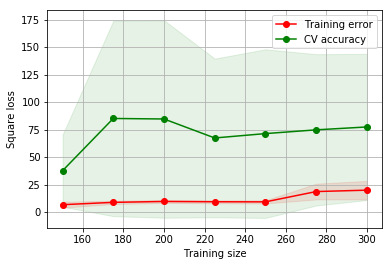Linear regression look unstable here. The risk estimates oscillate between $40$ and $80$ and have a huge variance.

We repeat the experiment without cross-validation so that we can extract the linear model $\bwhat$ learned by linear regression for each training set size

In :
coef_list = []

for s in range(150, 301, 25):
X_train, X_test, y_train, y_test = train_test_split(X, y, train_size=s, random_state=42)
learner.fit(X_train, y_train)
coef_list.append(learner.coef_)

coef_matrix = np.array(coef_list)

/home/nicolo/anaconda3/lib/python3.7/site-packages/sklearn/model_selection/_split.py:2026: FutureWarning: From version 0.21, test_size will always complement train_size unless both are specified.
FutureWarning)


Then, we use PCA to visually check how different the learned models are.

In :
pca = decomposition.PCA(n_components=2)
pca.fit(coef_matrix)
coef_pca = pca.transform(coef_matrix)

fig, ax = plt.subplots()
ax.set_xlim(-1.5, 1.5)
ax.set_ylim(-0.5, 0.5)
plt.scatter(coef_pca[:,0], coef_pca[:,1])

Out:
<matplotlib.collections.PathCollection at 0x7f6bd812cdd8>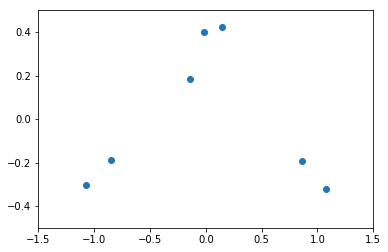In order to increase the stability of linear regression, we first use PCA to reduce the number of features and increase the bias.

In :
pca = decomposition.PCA(n_components=11)
pca.fit(X)

plt.title('PCA')
plt.plot(pca.singular_values_, label='Singular values')
plt.legend()
plt.show()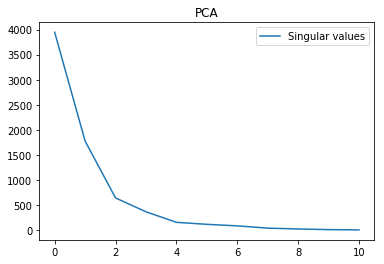We project all the datapoints on the six principal components. Then we check the learning curve again.

In :
pca = decomposition.PCA(n_components=6)
pca.fit(X)
X_pca = pca.transform(X)

In :
sizes = range(150, 301, 25)
train_size, train_score, val_score = learning_curve(learner, X_pca, y, train_sizes=sizes, cv=5, scoring=RMS)

In :
plt.title('PCA Linear Regression')
train_score_mean = -np.mean(train_score, axis=1)
train_score_std = np.std(train_score, axis=1)
val_score_mean = -np.mean(val_score, axis=1)
val_score_std = np.std(val_score, axis=1)
plt.grid()
plt.fill_between(sizes, train_score_mean - train_score_std,
train_score_mean + train_score_std, alpha=0.1,
color="r")
plt.fill_between(sizes, val_score_mean - val_score_std,
val_score_mean + val_score_std, alpha=0.1, color="g")
plt.plot(sizes, train_score_mean, 'o-', color="r",
label="Training error")
plt.plot(sizes, val_score_mean, 'o-', color="g",
label="CV risk estimate")
plt.legend()
plt.xlabel('Training size')
plt.ylabel('Square loss')
plt.show()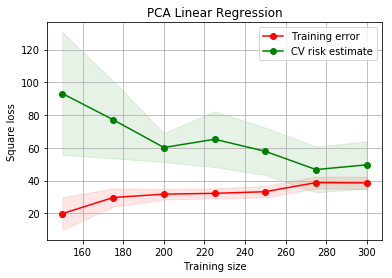The learning curve looks more stable now. We doublecheck by repeating the procedure to plot the spread of the vectors learned using increasing sizes of the training set.

In :
coef_list = []

for s in range(150, 301, 25):
X_train, X_test, y_train, y_test = train_test_split(X_pca, y, train_size=s, random_state=42)
learner.fit(X_train, y_train)
coef_list.append(learner.coef_)
coef_matrix = np.array(coef_list)

pca = decomposition.PCA(n_components=2)
pca.fit(coef_matrix)
coef_pca = pca.transform(coef_matrix)

/home/nicolo/anaconda3/lib/python3.7/site-packages/sklearn/model_selection/_split.py:2026: FutureWarning: From version 0.21, test_size will always complement train_size unless both are specified.
FutureWarning)

In :
fig, ax = plt.subplots()
ax.set_xlim(-1.5, 1.5)
ax.set_ylim(-0.5, 0.5)
plt.scatter(coef_pca[:,0], coef_pca[:,1])

Out:
<matplotlib.collections.PathCollection at 0x7f6bd805aba8>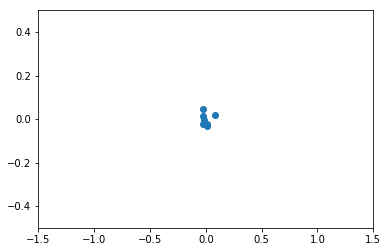As the plot shows, the learned models are a indeed more stable after PCA.

## Ridge Regression¶

A better way to increase the bias in linear regression, and thus make the model more stable, is by introducing a regularizer in the ERM functional.

In other words, instead defining $\bwhat$ by $$\bwhat = \argmin_{\bw\in\R^d} \norm{S\bw-\by}^2$$ we use the regularized form, also known as Ridge Regression, $$\bwhat = \argmin_{\bw\in\R^d} \norm{S\bw-\by}^2 + \alpha\norm{\bw}^2$$ where $\alpha \ge 0$ is the regularization parameter. When $\alpha$ is zero we recover the standard linear regression solution. When $\alpha$ is very large, the solution $\bwhat$ becomes the zero vector. Hence $\alpha$ can be used to control the bias of the algorithm.

Similarly to before, we have that $$\nabla\norm{S\bw-\by}^2 + \alpha\norm{\bw}^2 = 2S^{\top}(S\bw-\by) + 2\alpha\bw$$
Hence, the gradient vanishes for $\bw = \big(\alpha I + S^{\top}S\big)^{-1}S^{\top}\by$. Note that we do not have to worry anymore about the invertibility of $S^{\top}S$. Indeed, if $\lambda_1 \ge\cdots\ge \lambda_d \ge 0$ are the eigenvalues of $S^{\top}S$, the eigenvalues of $I + S^{\top}S$ are simply $1+\lambda_1 \ge\cdots\ge 1+\lambda_d > 0$.

We start by investigating the sensitivity of the CV risk estimate to the choice of $\alpha$.

In :
alpha_vals = np.linspace(0.01,2.0,20)
train_score, val_score = validation_curve(Ridge(normalize=True), X, y, 'alpha', alpha_vals, cv=5, scoring=RMS)

In :
plt.title('Ridge Regression')
train_score_mean = -np.mean(train_score, axis=1)
train_score_std = np.std(train_score, axis=1)
val_score_mean = -np.mean(val_score, axis=1)
val_score_std = np.std(val_score, axis=1)
plt.grid()
plt.fill_between(alpha_vals, train_score_mean - train_score_std,
train_score_mean + train_score_std, alpha=0.1,
color="r")
plt.fill_between(alpha_vals, val_score_mean - val_score_std,
val_score_mean + val_score_std, alpha=0.1, color="g")
plt.plot(alpha_vals, train_score_mean, color="r",
label="Training error")
plt.plot(alpha_vals, val_score_mean, color="g",
label="CV risk estimate")
plt.legend()
plt.xlabel('Alpha')
plt.ylabel('Square loss')
plt.show()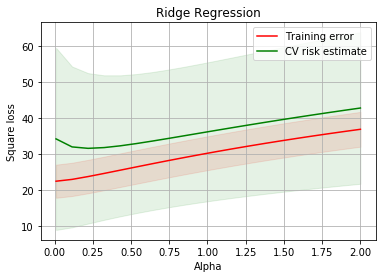We see that overfitting essentially disappears for $\alpha > 0.5$, and bias progressively increases. The variance of the CV risk estimate remains high though.

With normalized data (as performed by the flag normalize=True), the choice $\alpha = 1$ is often reasonable.

We now compare the learning curves of PCA Linea Regression and Ridge Regression.

In :
lin_reg = LinearRegression(normalize=True)

sizes = range(150, 301, 25)
l_train_size, l_train_score, l_val_score = learning_curve(lin_reg,
X_pca, y, train_sizes=sizes, cv=5, scoring=RMS)
r_train_size, r_train_score, r_val_score = learning_curve(Ridge(normalize=True),
X_pca, y, train_sizes=sizes, cv=5, scoring=RMS)

In :
plt.title('PCA Linear Regression vs. Ridge Regression')
l_val_score_mean = -np.mean(l_val_score, axis=1)
l_val_score_std = np.std(l_val_score, axis=1)
r_val_score_mean = -np.mean(r_val_score, axis=1)
r_val_score_std = np.std(r_val_score, axis=1)
plt.grid()
plt.fill_between(sizes, l_val_score_mean - l_val_score_std,
l_val_score_mean + l_val_score_std, alpha=0.1,
color="r")
plt.fill_between(sizes, r_val_score_mean - r_val_score_std,
r_val_score_mean + r_val_score_std, alpha=0.1, color="g")
plt.plot(sizes, l_val_score_mean, 'o-', color="r",
label="PCA Linear Regression")
plt.plot(sizes, r_val_score_mean, 'o-', color="g",
label="Ridge Regression")
plt.legend()
plt.xlabel('Training size')
plt.ylabel('CV risk estimate')
plt.show()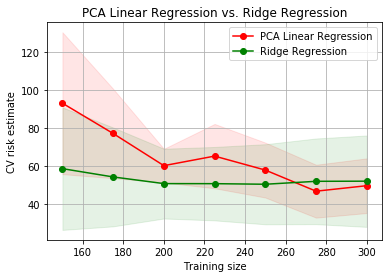We see that, despite a large variance in the CV risk estimate, Ridge Regression is better than PCA Linear Regression, especially in the small training set regime.